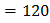# In a copper voltmeter , if the current (and timevariations of the type as shown in figure, the mass deposited in 30 min is [Atomic weight of copper is 63.5 and Faraday constant is 96500 C per g equivalent] a) 0.078 g b) 0.054 g c) 0.039 g d) 0.0195 g

## Question ID - 150389 :- In a copper voltmeter , if the current (and timevariations of the type as shown in figure, the mass deposited in 30 min is [Atomic weight of copper is 63.5 and Faraday constant is 96500 C per g equivalent] a) 0.078 g b) 0.054 g c) 0.039 g d) 0.0195 g

3537

 (c) Charge flowing in 30 min,area under graphCg
 Identify the operation performed by the circuit given in the figure.a) NOT b) AND c) OR d) NAND